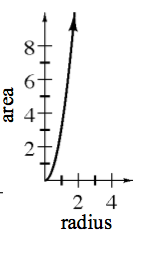### Home > A2C > Chapter 1 > Lesson 1.2.1 > Problem1-73

1-73.

Consider circles of different sizes. Create multiple representations of the function with inputs that are the radius of the circle and outputs that are its area.

How do you find the area of a circle? (If you forgot, try searching for the formula on the Internet.)

Which variable is independent, the radius or the area?

 $x$ $y$ $0$ $1$ $2$ $3$ $4$ $0$ $\pi$ $4\pi$ $9\pi$ $16\pi$